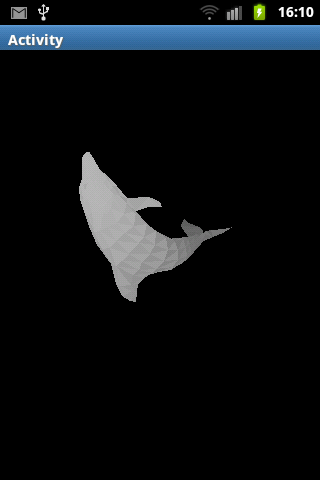# Share My CreationSimple 3D Wireframe Object

Hello! This is one of my first B4A apps I have made. This app allows you to display a set of nodes, faces in wireframe. You can also rotate the object by dragging your finger across the screen. It was a little difficult to make, mainly cause I don't know the little differences between B4A and .Net Basic.

Pretty sloppy code, only took me a few hours.
http://pastebin.com/RYpFTHDu

Uses RotateX to rotate Y-Nodes, Uses RotateY to rotate X-Nodes. Loops through the nodes in the array of objects held by the object structure and edit their values. Pretty simple. Although, I don't know much trig or geomety, im only in pre-algebra. If anyone wants to help me shade the object, I already made faces instead of edges, but I'm only in pre-algebra so I don't know much trigonometry or geometry and I can't really figure it outThank you•wonder, ilan and Erel

#### lucas heer

##### New Member
Longtime User
I finally got shading to work, using Painters Algorithm. Which is basically adding up all the y-values and ordering the nodes or vertices from lowest to highest and displaying back sides first. Its a little glichy, on the cube, the 2 sides other than the most-facing front side switch colors. Still trying to figure out whyIt works in .net though. I used list in .net vb to organize Z values from low to high so that might be why. ThanksStill sloppy code : http://pastebin.com/XcuVFkJ0•Mashiane and Peter Simpson

#### sorex

##### Expert
Longtime User
@lucas heer : there are several ways to get it done right, but z-sorting is indeed the way to go to get some neat effects.

here is a screenshot of what I wrote 3 years ago as a sort of Android speed test to see how fast my low end phone was.
ran quite well knowing that it (3DS dolphin) contains 562 polygons (285 vertices) and used only the core drawing routines.

Using openGL would be a lot faster but I never got to that so far.#### Peter Simpson

##### Expert
Longtime User
Share the source @sorex#### sorex

##### Expert
Longtime User
this is the most important part of the routine which recalculates vertices positions

B4X:
``````For n=0 To vertices.Size-1
Dim co As coord
v=vertices.Get(n)
x3d = v.x
y3d = v.y
z3d = v.z
ty = ((y3d * Cos(vx)) - (z3d * Sin(vx)))
tz = ((y3d * Sin(vx)) + (z3d * Cos(vx)))
tx = ((x3d * Cos(vy)) - (tz * Sin(vy)))
tz = ((x3d * Sin(vy)) + (tz * Cos(vy)))
ox = tx
tx = ((tx * Cos(vz)) - (ty * Sin(vz)))
ty = ((ox * Sin(vz)) + (ty * Cos(vz)))
co.x  = (500* (tx) / (distance - (tz))) +135
co.y  = (135 - (500 * ty) / (distance - (tz)))
co.z=tz
c=( ( tz+30)*5)
color=(c*256*256)+(c*256)+c
co.col=color
Next``````

#### Peter Simpson

##### Expert
Longtime User
I was not expecting that @sorex#### Douglas Farias

##### Expert
Longtime User
For n=0To vertices.Size-1Dim co As coord
v=vertices.Get(n)
x3d = v.x
y3d = v.y
z3d = v.z
ty = ((y3d * Cos(vx)) - (z3d * Sin(vx)))
tz = ((y3d * Sin(vx)) + (z3d * Cos(vx)))
tx = ((x3d * Cos(vy)) - (tz * Sin(vy)))
tz = ((x3d * Sin(vy)) + (tz * Cos(vy)))
ox = tx
tx = ((tx * Cos(vz)) - (ty * Sin(vz)))
ty = ((ox * Sin(vz)) + (ty * Cos(vz)))
co.x = (500* (tx) / (distance - (tz))) +135
co.y = (135 - (500 * ty) / (distance - (tz)))
co.z=tz
c=( ( tz+30)*5)
color=(c*256*256)+(c*256)+c
co.col=colorThis is b4a xD????????

#### lucas heer

##### New Member
Longtime User
this is the most important part of the routine which recalculates vertices positions

B4X:
``````For n=0 To vertices.Size-1
Dim co As coord
v=vertices.Get(n)
x3d = v.x
y3d = v.y
z3d = v.z
ty = ((y3d * Cos(vx)) - (z3d * Sin(vx)))
tz = ((y3d * Sin(vx)) + (z3d * Cos(vx)))
tx = ((x3d * Cos(vy)) - (tz * Sin(vy)))
tz = ((x3d * Sin(vy)) + (tz * Cos(vy)))
ox = tx
tx = ((tx * Cos(vz)) - (ty * Sin(vz)))
ty = ((ox * Sin(vz)) + (ty * Cos(vz)))
co.x  = (500* (tx) / (distance - (tz))) +135
co.y  = (135 - (500 * ty) / (distance - (tz)))
co.z=tz
c=( ( tz+30)*5)
color=(c*256*256)+(c*256)+c
co.col=color
Next``````

I was wondering what your coord structure looked like, and sorry for noobiness, I'm only at pre-algebra math level lol, but what are 135 and 500 for? Also, how would u go about calculating Z-locations for ordering the order of drawing faces? Thanks#### sorex

##### Expert
Longtime User
This is b4a xD????????

sure, it's just math@lucas heer :

the coord is just a type

B4X:
``Type coord (x As Int, y As Int,z As Int, col As Int)``

I sum the 3 z values and divide it by 3 to get the avarage and sort on that.

the odd values is to center/scale it. the inital data looks like

B4X:
``````2.229 9.601 0.4095
1.96 9.639 2.145e-005
3.905 11.39 2.389e-005
4.288 11.33 0.3073
5.567 12.19 2.486e-005
5.734 12.04 0.1793
7.156 12.31 2.486e-005
7.241 12.1 0.06399
8.06 12.07 2.486e-005
7.182 9.113 0.4991
7.156 10.02 2.194e-005
7.689 8.768 2.047e-005``````

so that would be a very tiny dolphin if you stick to thatAndroid Question Android 3d Viewer
Replies
4
Views
723
Replies
15
Views
2K
Replies
10
Views
6K
Android Example 3D Spinning Cube
Replies
12
Views
6K
Replies
17
Views
9K